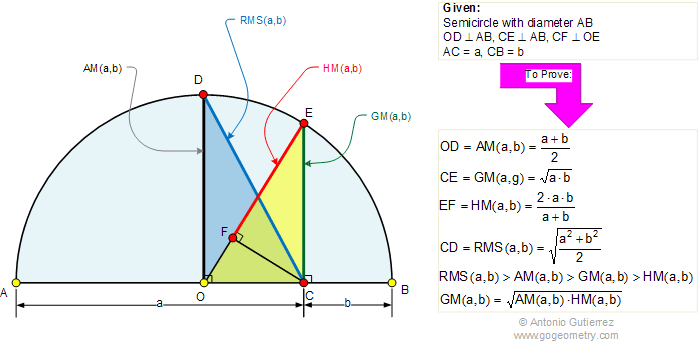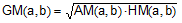# Arithmetic Mean, Geometric Mean, Harmonic Mean, Root Mean SquareThe figure above shows a semicircle with diameter AB and center O. C is a point on AB, CE and OD are perpendicular  to AB, and CF is perpendicular to OE. If AC = a and CB = b, prove that: OD is the arithmetic mean of a and b; AM(a,b). CE is the geometric mean of a and b; GM(a,b). EF is the harmonic mean of a and b; HM(a,b). CD is the root mean square of a and b; RMS(a,b) Inequality: RMS (a,b) > AM (a,b) > GM (a,b) > HM (a,b).

 Home | Search | Geometry | Geometric Mean | Harmonic Mean | RMS Index | Perpendicular Chords | Post a comment | Email | by Antonio Gutierrez Last updated: Mar 9, 2022